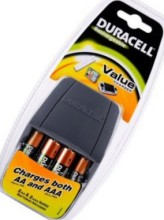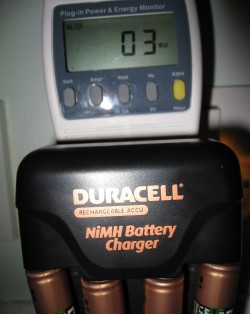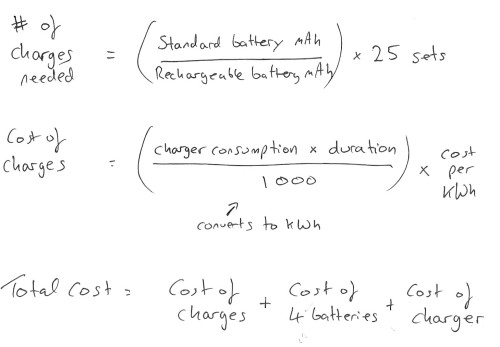# Rechargeable Batteries vs Standard Batteries

### Does it make sense to get rechargeable batteries?

For this calculation, we’re going to assume our electronic device needs 4 x AA batteries.

### Standard Batteries

We’ll use Duracell AA 1.5V Alkaline batteries rated at 2200mAh. Cost per 4 pack is £3.50 at Tesco

Total cost: 25 packs at £3.50 = £87.50

###Rechargeable Batteries

Rather that the older and less reliable NiCad batteries, we found a handy charger from Duracell that uses NiMH (the type of battery used in a mobile phone). The charger is the Duracell Rechargeable Value Charger. This comes with a pack of 4 AA 1.2V Alkaline batteries rated at 1800mAh.

The charger, plus the 4 batteries costs £15 at Tesco – Duracell Value Charger Pack

### The Maths

As the mAh on the rechargeable batteries is lower, we need to find out how many charging cycles will be needed to get the same consumption as 25 packs of Alkaline batteries.

We then need to work out the cost per charging cycle. On our power meter, we see that the charger draws 3 watts. Recommended charge time is listed on the charger as 6 hours. 18 watts per charging cycle.If we multiply this by the number of cycles, we get the total Watt Hour. Divide by 1000 to get kWh, then look on an electricity bill to get the cost of a kilowatt hour (12p per kWh with EDF at time of writing). Multiply the total kWh of the charges by the unit cost. Then add the cost of the charger and batteries. Here are Pete’s scribblings:### The Result?

Our Pete’s done the maths. For charging 4 x AA batteries 30 times, you’ll pay less than 10p. With the cost of the rechargeable batteries and the charger, the total is  15.07, compared to  87.50 for the Alkaline battery equivalent